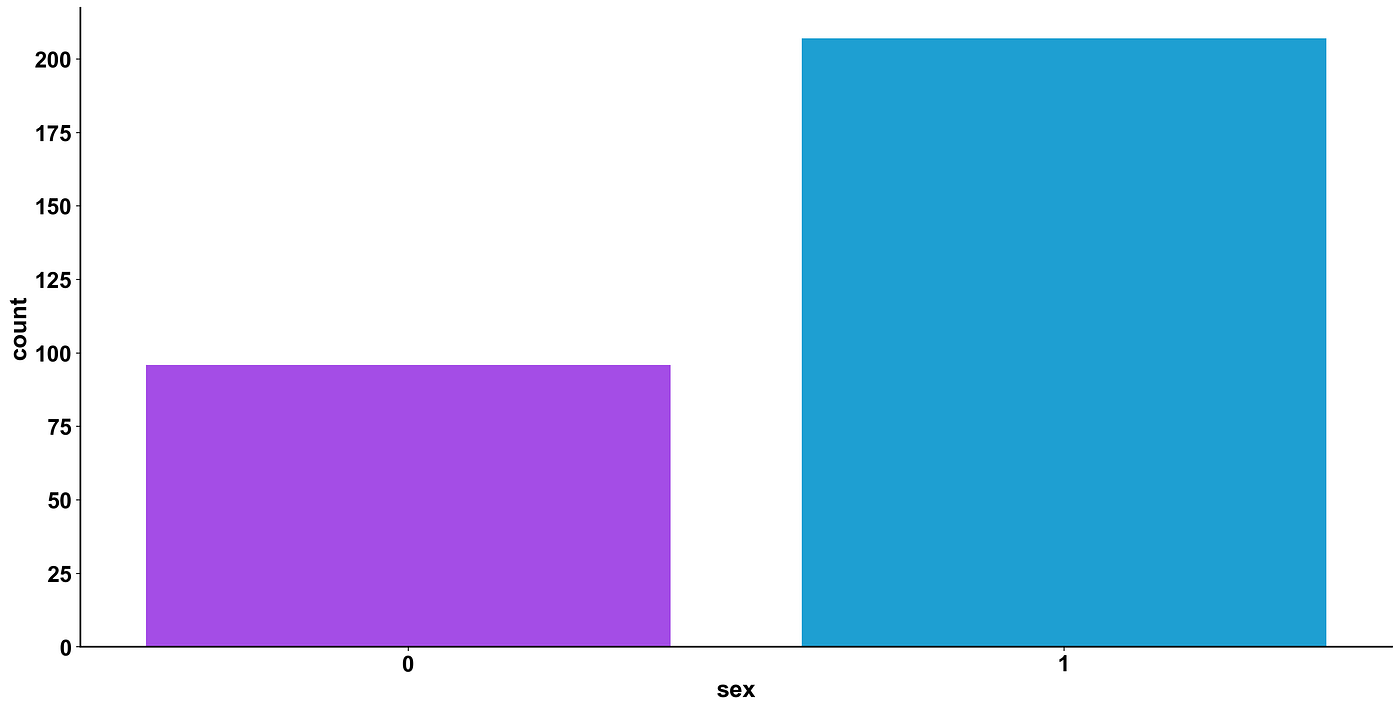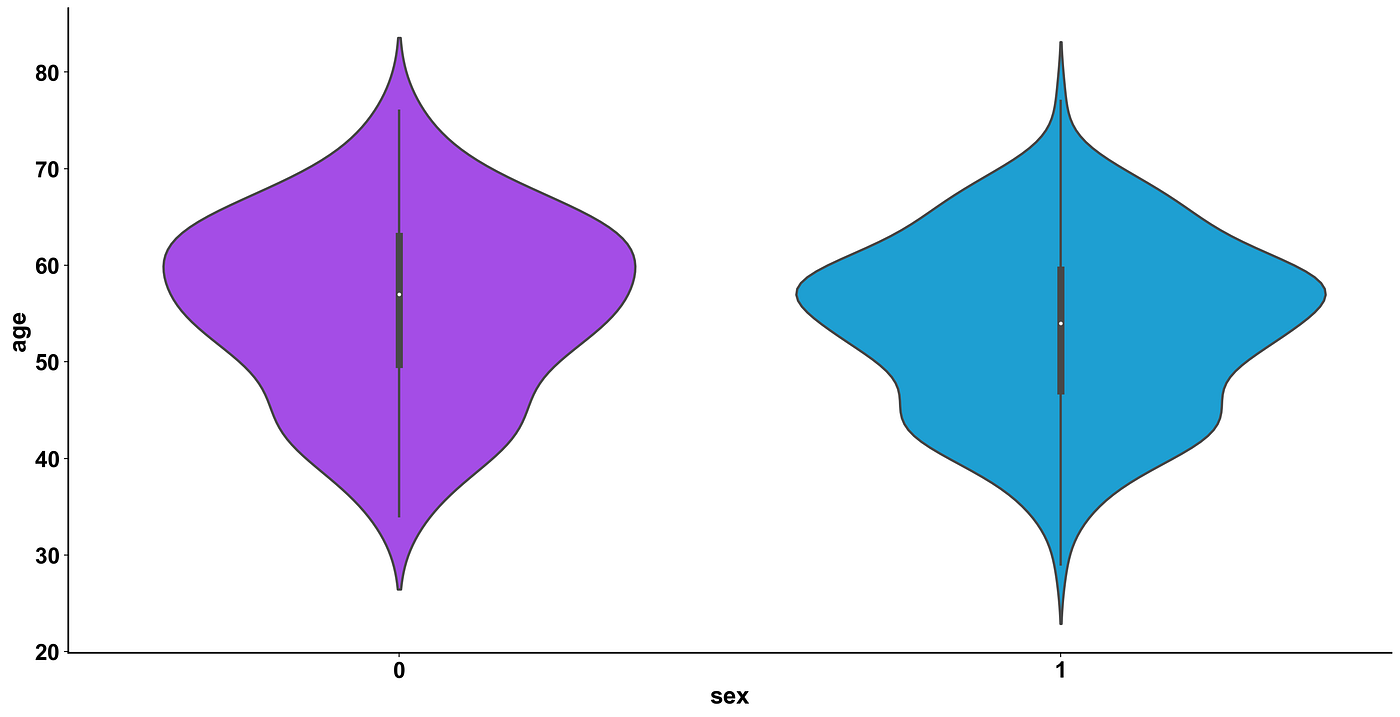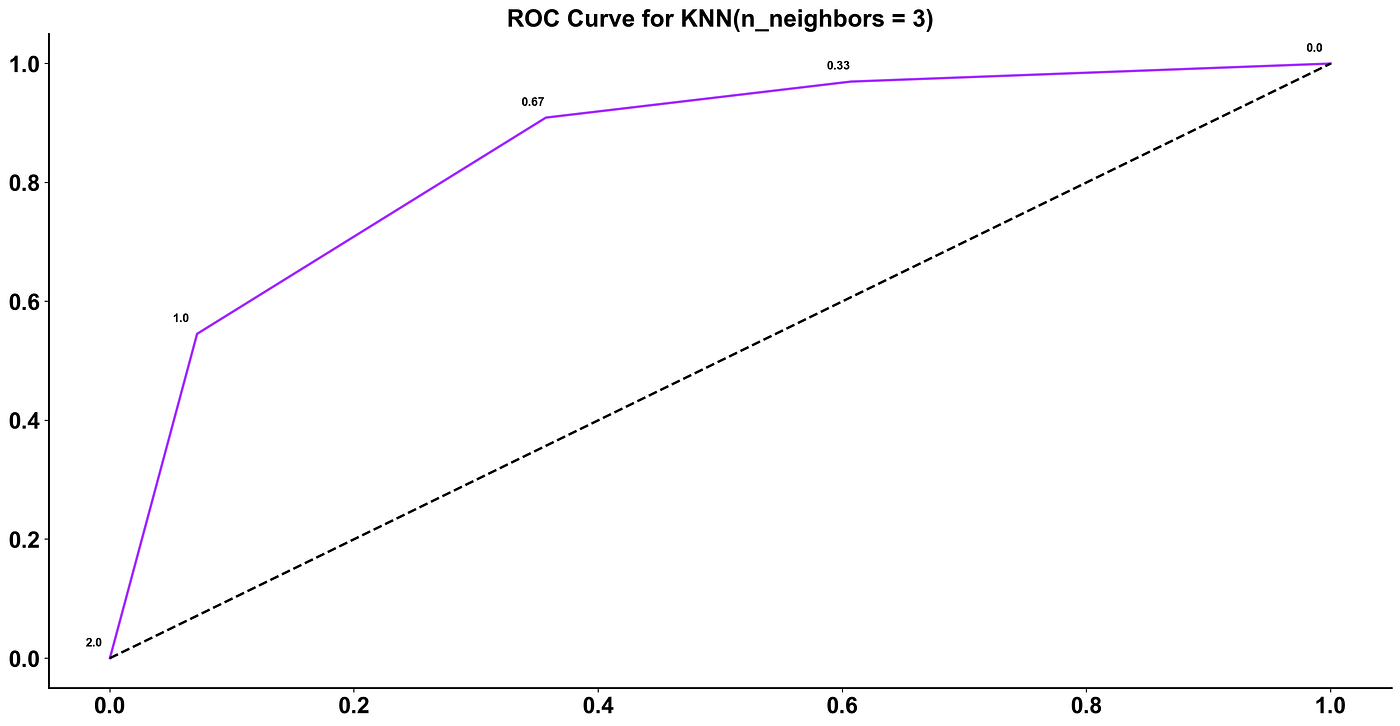# Are you susceptible to a heart attack? A Machine Learning approachPhoto by Tamanna Rumee on Unsplash

Among the workshops proposed in Datern’s data science course, one in particular impressed me.
The task, as the title hints, is to decide whether a patient has a heart disease or not, based on his or her physiology.
In the rest of the article, I’ll present my findings and the method I used to achieve them.

The dataset used contains 303 total patients, with 14 variables:
age,
sex,
cp (chest pain type),
trestbps (resting blood pressure),
chol (cholesterol),
fbs (fasting blood sugar),
restecg (resting ecg),
thalach (maximum heart rate achieved),
exang (exercise induced angina),
oldpeak (ST depression induced by exercise relative to rest),
slope (the slope of the peak exercise ST segment),
ca (number of major vessels (0–3) colored by flourosopy),
thal,
target (heart attack or not)

Let’s explore the data further

This count-plot shows the proportion of men to women examined in the dataset:0 denotes female, 1 denotes men

We can see that that women are 98, men 205. This could result in a stronger accuracy in predicting the diagnosis of the disease in male patients.

This violin-plot instead looks at the age distribution among men and women:0 denotes female, 1 denotes men

It shows that female are more homogenous in age compared to male and they tend to be older.

This correlation matrix analyses the correlations between the variables:

We can see that the feature most correlated to the target seems to be “exang” (exercise induced angina). Also, we observe that there isn’t evidence of multicolinearity between the features.

We now proceed to build a K Nearest Neighbours model to predict the “target”, i.e. whether the patient has a heart disease (1) or not (0).

After placing each observation in a N-Dimensional plane, where N is the number of the features considered, KNN determines the class of unknown data by considering its K nearest neighbours. Each of them has a “vote”, and votes for the class they belong to. The class that has more votes at the end of the voting will be the class of the unknown observation.

In the following portion of code, we create the feature matrix X and the target vector y. Then, we scale the data. That’s crucial for knn, since it relies on a notion of distance. We achieve this with sklearn.preprocessing.StandardScaler.
Then we split the data in two parts: train and test (20% of the data). This is to avoid overfitting.

`# Create feature and target arraysy = df["target"].valuesX = df.drop(["target"], axis = 1)#Scaling - crucial for knnfrom sklearn.preprocessing import StandardScalerss = StandardScaler()X = ss.fit_transform(X)from sklearn.model_selection import train_test_split# Split into training and test setX_train, X_test, y_train, y_test = train_test_split(X, y, test_size = 0.2, random_state=42, stratify=y)`

In the following portion of code, we create a knn object with k=3. We train it with the .fit method and produce results with the .predict method. After all of this, accuracy will be printed out.

`from sklearn.neighbors import KNeighborsClassifier# Create a k-NN classifier with 3 neighborsknn1 = KNeighborsClassifier(n_neighbors = 3)# Fit the classifier to the training dataknn1.fit(X_train,y_train)# Print the accuracyprint(knn1.score(X_test, y_test))`

We get an accuracy of 79%. Let’s try some other values for k and try to improve this accuracy. This is the task of the next code snippet.

`# Setup arrays to store train and test accuraciesneighbors = np.arange(1, 16)train_accuracy = np.empty(len(neighbors))test_accuracy = np.empty(len(neighbors))# Loop over different values of kfor i, k in enumerate(neighbors):    # Setup a k-NN Classifier with k neighbors: knn    knn = KNeighborsClassifier(n_neighbors = k)    # Fit the classifier to the training data    knn.fit(X_train,y_train)        #Compute accuracy on the training set    train_accuracy[i] = knn.score(X_train, y_train)    #Compute accuracy on the testing set    test_accuracy[i] = knn.score(X_test, y_test)`

Now let’s plot the accuracy for each k

We see that the best accuracy is achieved when k = 12, but this could cause overfitting, so we should also consider other metrics to determine what value of k should be used.
For this reason, let’s plot a ROC (Receiver Operating Characteristic) curve. It is the plot between the TPR (y-axis) and FPR (x-axis). Since our model classifies the patient as having heart disease or not based on the probabilities generated for each class, we can decide the threshold of the probabilities as well. Let us generate a ROC curve for our model with `k = 3`.Little numbers above each vertex is the threshold considered.

The area with the curve and the axes as the boundaries is called the Area Under Curve (AUC). It is this area which is considered a sign of a good model. With this metric ranging from 0 to 1, we should aim for a high value of AUC. Models with a high AUC are known as models with good skill.

AUC for this model is 85%. It means that it will be able to distinguish the patients with heart disease and those without 85% of the time.

Another diagnostic tool is PRC (Precision-Recall curve). Again, it shows us precision and recall for different values of the threshold and we should aim to maximise the area under the curve.

For this model, AUC of PRC is 88%

We are now able to correctly predict whether new patients have a heart disease or not 85% of the times. Certainly an helpful diagnostic tool for doctors.

We should also remember that the model is biased by overfitting, so the true accuracy could be different than the value we obtained, and it also depends on the portion of data that the model has been trained on.

This is only the beginning of the project. Next steps would include trying different train/test split ratios, different kinds of distance, different combinations of features, etc…

Thank you for your time.

--

--